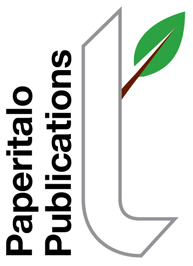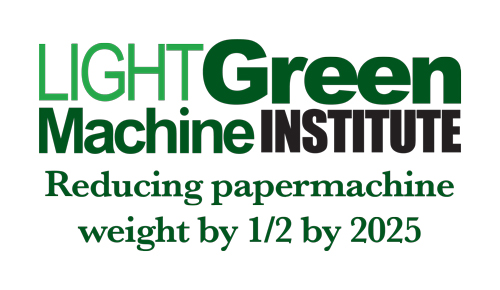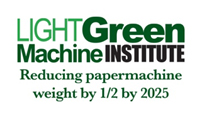The Light Green Machine InstituteSeptember 2019: The Optimization Problem--Clues to Solution, Part 2By Jim Thompson   Where we left things last month.... 1. We discussed encapsulating 2-D areas. 2. We derived the formulas to show a circular area is the most economical way (from a perimeter consideration) to encapsulate a given area.  In our example, starting with a radius of 3 units, we found a square can require 13% more perimeter wall than a circle. Further, an extreme rectangle (1 unit wide x 28.26 units long--to match the area encapsulated by our circle of 3 units radius) is 213% more costly, in terms of perimeter walls, than a circle. Now, if we move to 3-Dimensions (for all paper machine buildings are 3-Dimensions) our theoretical proxy is a sphere. Now we are talking about volume.  In fact, anyone who has visited a paper machine building subconsciously knows when we enter a paper machine building that it is a voluminous structure, unlike a football or baseball field, which in their static forms are essentially two dimensional structures (player dynamics make them 3-D). Some well-known paper machine cost estimators use volume as their first cut when they compare one machine's cost to another's. The volume of a sphere is (4/3) x pi x r^3. In our example, with a radius of 3 units, the volume is (4/3) x pi x 3 x 3 x 3= 113.09734 cubic units. The surface area of a sphere is 4 x pi x r^2.  So, in our case, the surface area is 4 x pi x 3 x 3 = 113.09734 square units. Now, if we attempt to create a cube containing 113.09734 units, we will find it is 4.84 units on a side (113.09734^(1/3)). Then it follows each side is 4.84^2 = 23.4 square units.  Since a cube has six sides, the total surface area is 6 x 23.4 = 140.55 square units.  Thus, a cube requires a surface area 24% larger than that of a sphere to encapsulate the same volume. Check my math and forward any corrections, please! To be continued...